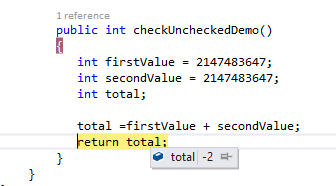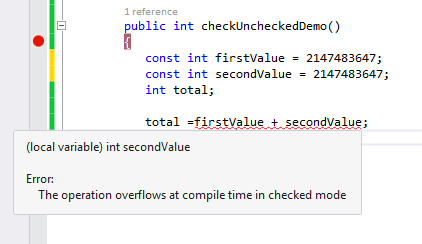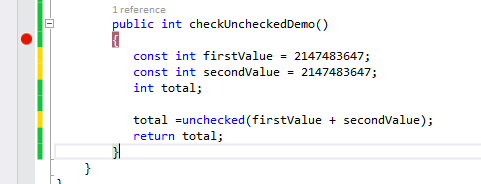© 2021 - First Crazy Developer (Abhishek Kumar)

###What is the use of "checked" & "unchecked" keyword in C#

Today we will discuss the use of "checked" and "unchecked" keywords and try to understand the importance of these keywords in C#.

We will start with some scenario then come to the point. Tell me what is the maximum value of int in C#?

1. Console.WriteLine(int.MaxValue); // 2147483647

When we will execute the above code, we will get the maximum value of int in c#. The answer of maximum value of int in C# is "2147483647".

Now look the following code:

1. public int checkUncheckedDemo ()
2. {
3. int firstValue = 2147483647;
4. int secondValue = 2147483647;
5. int total = firstValue + secondValue;
7. }

In above code we defined two int variables firstValue & secondValue and assigned the maximum int value into both variables. Now what am I trying to assign the addition of both variables into another integer value. Now what  happen if we execute the above code:

1.) It will not compile
2.) It will throw runtime error

I am dam sure most of coder doesn't have any idea about the final output. Now we compile the above code. The code is successfully compiled. So first answer is false. Now we execute the code and surprised, it didn't throw any runtime error( I am sure most developer had second option).What you think the answer is third option?

If we print the "total", what we get? Surprised, third option is also false. Curiosity come into mind, what is the output?

We get some weird value in total.The above code didn't match with any expected result. Because both variable are contain maximum value and when we try to store that value into another int variable that overflowed by total and now total have weird value.

If we write the above types of code in our project, not able to track these error. Here we need like some overflow types of exception so we can handle & track that.

Now the "checked" keyword comes into the frame. We can handle this scenario with "checked" keyword.

So, "checked" keyword what is does it, it actually says that if right hand side data push the over limited data into left hand side will throw up exception.

Look the updated code:

1. public int checkUncheckedDemo ()
2. {
3. int firstValue = 2147483647;
4. int secondValue = 2147483647;
5. int total =checked(firstValue + secondValue);
7. }

In above code now we put the "checked" keyword before pushing the data from right hand side into left hand side data type. Now if we execute the code we get OverflowException.

The "checked" keyword is used in scenario where we want to ensure that our left hand side data type not overflowed. With the help of try catch we can handle this situation in our code.

Look The following code:

1. public int checkUncheckedDemo ()
2. {
3. int firstValue = 2147483647;
4. int secondValue = 2147483647;
5. int total;
6. try
7. {
8. total = checked(firstValue + secondValue);
9. }
10. catch (OverflowException ex)
11. {
12. total = 0;
13. Console.WriteLine(ex.Message); // Arithmetic operation resulted in an overflow.
14. }
16. }

In above code we handled the scenario and could easily track these exception.

I hope we understood the importance of checked keyword in C#.

What is the use of unchecked keyword?

unchecked keyword has almost the same way like the default behaviour. If I am saying that unchecked keyword has same way as default behaviour then, why Microsoft introduce that? That’s why I said almost same not hundred percent our code?

If we talk about coding in C#, there are some interesting point, look the following code:

1. public int checkUncheckedDemo ()
2. {
3. const int firstValue = 2147483647;
4. const int secondValue = 2147483647;
5. int total;
6. total = firstValue + secondValue;
8. }When we try to compile the above code we get compile time error. Because our compiler check the overflow exception with const type data. If we want to avoid this overflow type exception, use unchecked keyword here.

1. public int checkUncheckedDemo ()
2. {
3. const int firstValue = 2147483647;
4. const int secondValue = 2147483647;
5. int total;
6. total =unchecked( firstValue + secondValue);
8. }Now if we try to compile the code, all code compiled successfully.

So finally we can say that, we will use the checked keyword if we want to ensure that no overflow happening in our code. And if we want to bypass the default behaviour of const type data we will use the unchecked keyword.

I hope we enjoyed this post, where we tried to understand that what the use is  of checked & unchecked keywords in C#.Home Page 16 July 2015